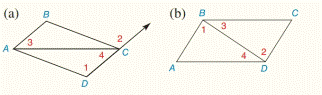Chapter 2.CR, Problem 1CRElementary Geometry For College St...

7th Edition
Alexander + 2 others
ISBN: 9781337614085

Solutions

Chapter
SectionElementary Geometry For College St...

7th Edition
Alexander + 2 others
ISBN: 9781337614085
Textbook Problem

If m ∠ 1 = m ∠ 2 , which line segments are parallel?To determine

a)

To find:

The parallel lines.

Explanation

Given:

The given statement is,

m1=m2

Figure (1)

Approach:

The given statement is,

m1=m2

So,

12

1 and 2 are two congruent angles

To determine

(b)

To find:

The parallel lines.

Still sussing out bartleby?

Check out a sample textbook solution.

See a sample solution

The Solution to Your Study Problems

Bartleby provides explanations to thousands of textbook problems written by our experts, many with advanced degrees!

Get Started

For what values of x is f continuous? f(x)={0ifxisrational1ifxisirrational

Single Variable Calculus: Early Transcendentals, Volume I

Fill in each blank: 3mi=rods

Elementary Technical Mathematics

Prove 227=01x4(1x)41+x2dx

Calculus of a Single Variable

True or False: If the three limits exist, then .

Study Guide for Stewart's Single Variable Calculus: Early Transcendentals, 8th